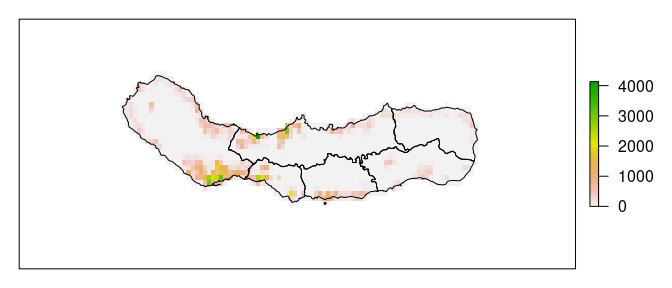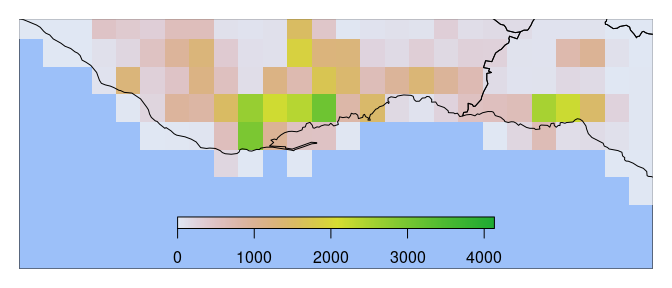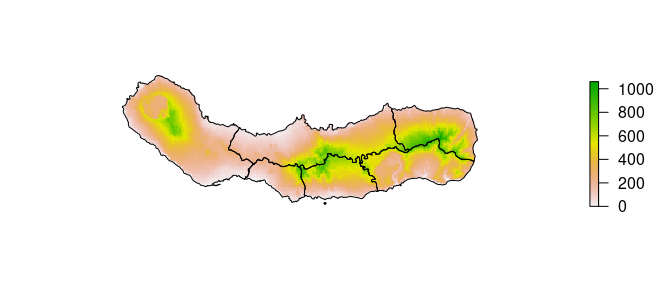# 1. Summarizing gridded population data

## Introduction

This vignette describes the use of exactextractr to summarize population and elevation data from the Gridded Population of the World and EU-DEM datasets. The exactextractr package includes samples of both of these datasets, cropped to the extent of São Miguel, the largest and most populous island of the Azores archipelago.

This example uses the following packages:

library(dplyr)
library(exactextractr)
library(raster)
library(sf)

To begin, we load the population count file from GPW. This raster provides the total population in each pixel for the calendar year 2020. On top of the population grid, we plot boundaries for the six municipalities, or concelhos, into which the island is divided. We can see that the population is concentrated along the coastlines, with smaller communities located in volcanic calderas inland.

pop_count <- raster(system.file('sao_miguel/gpw_v411_2020_count_2020.tif',
package = 'exactextractr'))
package = 'exactextractr'),
quiet = TRUE)
plot(pop_count, axes = FALSE)
plot(st_geometry(concelhos), add = TRUE)## Calculating total population

Because the population count raster has been cropped and contains no land area outside of São Miguel, we can calculate the total population of the island using the cellStats function from the raster package.

cellStats(pop_count, 'sum')
#>  145603

### Calculating population from the GPW population count file

We might also attempt to use exact_extract with the population count raster to see how the population is divided among concelhos:

exact_extract(pop_count, concelhos, 'sum', progress = FALSE)
#>  14539.875  4149.851 66866.711  5293.968 31920.496  9093.449

The result is a vector with one entry for each feature in concelhos. The order of the result is consistent with the input features, so we can assign the result of exact_extract to a new column in concelhos if desired.

To calculate the populations, we used fun = 'sum', where 'sum' is a named summary operation recognized by exactextractr. A full list of supported operations can be found in the function documentation for exact_extract. If none of the named operations is suitable, we can set fun equal to an R function such as function(pixel_value, coverage_fraction) sum(pixel_value * coverage_fraction). However, the named operations are generally faster than R equivalents and use less memory when rasters or polygons are large.

To review the results more easily, we can use the append_cols argument to copy columns from the input sf object into the result of exact_extract. We also use some dplyr operations to add a column for the total population of all concelhos:

concelho_pop <- exact_extract(pop_count, concelhos, 'sum',
append_cols = 'name', progress = FALSE) %>%
rename(pop = sum) %>%
arrange(desc(pop)) %>%
bind_rows(summarize(., name = 'Total', pop = sum(pop)))

This produces the following table:

name pop
Ribeira Grande 31,920
Lagoa 14,540
Vila Franca do Campo 9,093
Povoação 5,294
Nordeste 4,150
Total 131,864

We might reasonably expect the total population to equal the value of 145,603 we previously obtained using cellStats, but it doesn’t. In fact, 9% of the population is unaccounted for in the concelho totals.

The cause of the discrepancy can be seen by looking closely at the densely populated Ponta Delgada region on the southern coast. Many of the cells containing population are only partially covered by the concelho boundaries, so some of the total population calculated by cellStats is missing from the totals.

plot.new()
plot.window(xlim = c(-25.75, -25.55), ylim = c(37.70, 37.77))
rect(par("usr"), par("usr"), par("usr"), par("usr"), col = "#9cc0f9")
plot(pop_count, add = TRUE, axes = FALSE, alpha = 0.8, horizontal = TRUE)
plot(st_geometry(concelhos), add = TRUE)### Calculating population from the GPW population density file

It turns out that we need a somewhat more complex solution to get accurate population counts when our polygons follow coastlines. Instead of using the population count raster, we bring in the population density raster, which provides the number of persons per square kilometer of land area in each pixel.

pop_density <- raster(system.file('sao_miguel/gpw_v411_2020_density_2020.tif',
package = 'exactextractr'))

To get a population count, we can multiply the population density by the area of each cell that is covered by the polygon. One way to do this is by providing the cell areas as a weighting raster and using a custom summary function. Weighted summary functions have the signature function(values, coverage_fractions, weights).

We can write one as follows:

concelho_pop2 <- exact_extract(pop_density, concelhos,
function(density, frac, area) {
sum(density * frac * area)
},
weights = raster::area(pop_density),
append_cols = 'name',
progress = FALSE)
#> Warning in CPP_exact_extract(x, x_ext, x_res, x_orig, weights, wkb,
#> default_value, : value raster implicitly disaggregated to match higher
#> resolution of weights

This produces the following table:

name pop
Ribeira Grande 35,935
Lagoa 15,702
Vila Franca do Campo 11,704
Povoação 5,965
Nordeste 4,513
Total 144,801

The total population obtained using this method is remarkably close (within 0.55%) to the expected value from cellStats.

While this solution works well for the sample data, it has a couple of disadvantages for larger data sets:

• calling raster::area(x) generates an in-memory raster of the same size as x. For a raster like GPW at 30 arc-second resolution, this would consume several gigabytes of memory.
• passing extracted raster values to a summary function written in R requires that exactextractr load all values associated with a given polygon into memory at once. This presents no problem when working with the concelho boundaries, but could cause excessive memory usage when working with large national boundaries.

An alternative formulation that resolves both of these problems uses the weighted_sum summary operation instead of an R function, and uses weights = 'area', which instructs exact_extract to compute its own cell areas based on the projection of pop_density.

exact_extract(pop_density, concelhos, 'weighted_sum', weights = 'area')

## Population-weighted statistics

Suppose that we are interested in calculating the average elevation of a residence in each of the six concelhos. Loading the EU-DEM elevation data for the island, we can see that each concelho is at least partly occupied by interior mountains, indicating that the results of a simple mean would be unrepresentative of the primarily coastal population.

elev <- raster(system.file('sao_miguel/eu_dem_v11.tif', package = 'exactextractr'))

plot(elev, axes = FALSE, box = FALSE)
plot(st_geometry(concelhos), add = TRUE)As in the previous section, we avoid working with the population count raster to avoid losing population along the coastline. We can formulate the population-weighted average elevation as in terms of population density and pixel areas as:

$\bar{x}_\mathrm{pop} = \frac{ \Sigma_{i=0}^n {x_ic_id_ia_i}}{\Sigma_{i=0}^n{c_id_ia_i}}$ where $$x_i$$ is the population of pixel $$i$$, $$c_i$$ is the fraction of pixel $$i$$ that is covered by a polygon, $$d_i$$ is the population density of pixel $$i$$, and $$a_i$$ is the area of pixel $$i$$.

If we are working with projected data, or geographic data over a small area such as São Miguel, we can assume all pixel areas to be equivalent, in which case the $$a_i$$ components cancel each other out and we are left with the direct usage of population density as a weighting raster:

exact_extract(elev, concelhos,  'weighted_mean', weights = pop_density)

What if pixel areas do vary across the region of our analysis?

One option is to create a scaled population count raster by multiplying the population density and the pixel area. For pixels that are partly covered by water, this inflates the pixel population such that we obtain the correct population when only the land area is covered by a polygon. This requires that we create and maintain a separate raster data set.

Another option is to create a RasterStack of pop_density and area(pop_density), and then write a summary function to handle the necessary processing. We use the summarize_df = TRUE argument to combine the elevation, population density, pixel area, and pixel coverage fraction into a single data frame that is passed to the summary function.

exact_extract(elev, concelhos,
function(df) {
weighted.mean(x = df$value, w = df$coverage_fraction * df$pop_density * df$area,
na.rm = TRUE)},
weights = stack(list(pop_density = pop_density,
area = area(pop_density))),
summarize_df = TRUE,
progress = FALSE)

This solution shares the same limitations with the previous example using an R summary function with raster::area(): we must precompute an area raster and store it in memory, and we must load all raster values intersecting a given polygon into memory at a single time.

A better solution is to use the coverage_area argument to exact_extract, which specifies that all calculations use the area of each cell that is covered by the polygon instead of the fraction of each cell that is covered by the polygon.

concelho_mean_elev <- exact_extract(elev, concelhos, c('mean', 'weighted_mean'),
weights = pop_density,
coverage_area = TRUE,
append_cols = 'name', progress = FALSE)

Here we also calculate the unweighted mean for comparison. We can see that the population-weighted mean elevation is substantially lower than the mean elevation in all concelhos.

name mean_elev pop_weighted_mean_elev
Lagoa 233.7098 76.87321
Nordeste 453.8504 192.47522
Ponta Delgada 274.4062 97.71867
Povoação 375.4573 170.45435
Ribeira Grande 312.0619 74.84953
Vila Franca do Campo 418.7338 92.20170